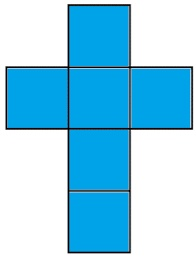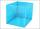# Cube edges

The sum of the lengths of the cube edges is 42 cm. Calculate the surface of the cube.

S =  73.5 cm2

### Step-by-step explanation:Did you find an error or inaccuracy? Feel free to write us. Thank you!## Related math problems and questions:

• Sum of the edgesThe sum of the lengths of all edges of the cube is 72 cm. How many cm2 of colored paper are we going to use for sticking?
• Edges or sidesCalculate the cube volume, if the sum of the lengths of all sides is 276 cm.
• Cube edgeDetermine the edges of the cube when the surface is equal to 37.5 cm square.
• Cube 5The surface of the cube is 15.36 dm2. How will change the surface area of this cube if the length of the edges is reduced by 2 cm?
• Cuboid surfaceDetermine surface area of cuboid if its volume is 52.8 cm cubic and length of the two edges are 2 cm and 6 cm.
• Cube 7Calculate the volume of a cube, whose sum of the lengths of all edges is 276 cm.
• CubeThe sum of all cube edges is 30cm. Find the surface area of the cube.
• Surface of cuboidFind the surface of the cuboid if its volume is 52.8 cm3 and the length of its two edges is 2 cm and 6 cm.
• Cube 1-2-3Calculate the volume and surface area of the cube ABCDEFGH if: a) /AB/ = 4 cm b) perimeter of wall ABCD is 22 cm c) the sum of the lengths of all edges of the cube is 30 cm.
• CubeThe sum of lengths of cube edges is 57 cm. What is its surface and volume?
• The cubeThe cube has a surface of 600 cm2. What is its volume?
• Cube V2SThe volume of the cube is 27 dm cubic. Calculate the surface of the cube.
• Cube 5The content area of one cube wall is 32 square centimeters. Determine the length of its edges, its surface and volume.
• The cubeThe cube has a surface area of 486 m ^ 2. Calculate its volume.
• CuboidCuboid has a surface of 516 cm2. Side a = 6 cm and b = 12 cm. How long is the side c =?
• Cube wallsFind the volume and the surface area of the cube if the area of one of its walls is 40 cm2.
• The surfaceThe cuboid's surface area is 1714 cm2, the edges of the base are 25 cm and 14 cm long. Find the area of the surface.